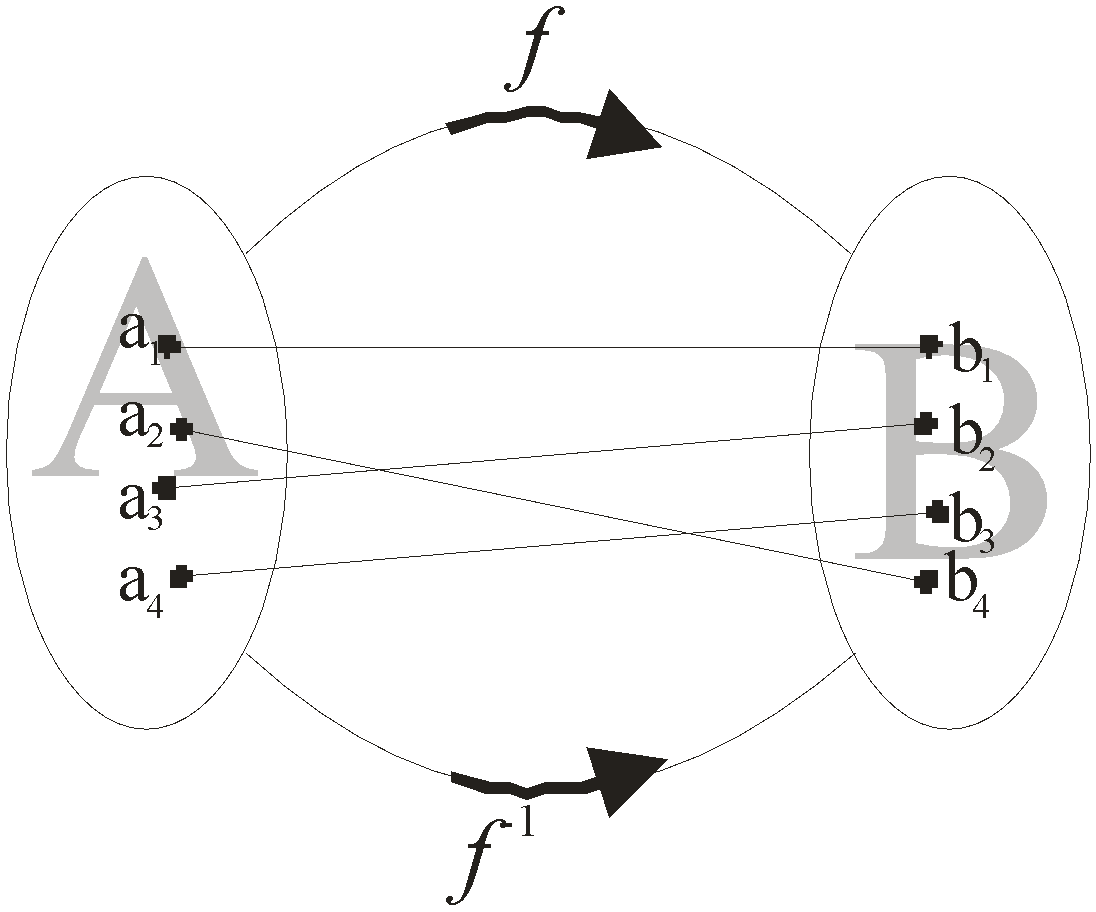BodhiAI
Nov. 11, 2019

#### Inverse of a Function & their Properties,Graph of Function & Their Applications:

Inverse of A Function

If f is a mapping from set A to set B, then the set A is called the domain and the set B is called the co-domain of the mapping f.

Now, if f:AB is bijective i.e. both one-to-one and onto then the mapping g or f-1 : BA is called the inverse mapping of f.

Reasoning: Let A and B be two sets and let f : A→B be a function.If we follow a rule in which elements of B are associated to their pre images, then we find that under such a rule there may be some elements in B which are not associated to elements in A. This happens when f is not an onto map. Therefore all elements in B will be associated to some elements in A if f is an onto map. Therefore an element in B will be associated to a unique element in A if f is an injective map.

It follows from the above discussion that if f: A→B is a bijection, we can define a new function from B to A which associates each element y∈B to its pre-image f-1(y)A. Such a function is known as the inverse of function f and is denoted by f-1.Important

The graph of an inverse function is symmetric in the line y = x.

Properties of Inverse Functions

P-1 The inverse of a bijection is unique.

P-2 The inverse of a bijection is also a bijection.

P-3 If f:A→B is a bijection and g: B→A is the inverse of f, then fog = IB and also gof = IA.

where, IA and IB are the identity functions on the sets A and B respectively.

P-4 If f:A→B and g:B→C are two bijections, then gof : A→C will also be a bijection and also (gof)-1 = f-1og-1

This is called Reversal Law of Inverses.

P-5 If f : AB and g : BA be two functions such that gof = IA and fog = IB. Then f and g are bijections and also g = f-1.